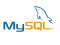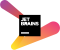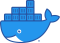🇷🇺|🇷🇸 Dmitriy Lezhnev
Software Developer
PHP/LEMP-stack/Go practitioner
Zend Certified Engineer
👔 On-Demand PHP DevelopmentFind me on the Internet

Sat, 20 Mar 2021

# # Evaluation Of Expressions In PHP (as of 7.4)

In programming languages an expression is something that can be evaluated to a single value. Like `5` is an expression that evaluates to integer 5, and `is_null(5)` is evaluated to boolean `false`. Expressions can be complex and contain many operators and function calls, also including parentheses that control the order of evaluation. See `abs(5 * (2 - 3))` evaluates to integer `5`.

When we write a complex expression, we expect that the result is predictable. We could write a test to confirm that, but also it'd good to have a clear understanding of how PHP interprets expressions. The skill of evaluating expressions with your eyes is quite handy in the code review process.

PHP is a translator which translates code written in PHP to code written in internal bytecode(opcode) language. The latter is then executed on the PHP's virtual machine. And as with any translator it needs to solve problems regarding the ambiguity of how to evaluate expressions, in which order, how to deal with side effects, how to deal with errors, and many more. As a language inspired by C, PHP inherits many ideas from it. Here I am going to overview some aspects of expression evaluation in PHP. Let's go!

## # Order Of Evaluation

PHP supports infix notation for expressions, which looks like `1 + 2`. Two operands and a binary operator between them. To support unary or ternary operators, PHP had to offer syntactical features. Unary operators are written like this `\$a++` or `--\$a`, while the only supported ternary operator looks like this: `\$a ? \$b : \$c` (or a short form `\$a ?: \$b` which looks like a normal binary operator). As you can see it forms as a combination of two binary operators. After all, infix notation is suited for operation with two arguments.

Infix notation is applied in math all over the world. It is also ambiguous in terms of interpretation. Expression `3 - 2 - 1` can be evaluated to `0` or to `2`. The order is determent by the "associativity" of the `-` operator. Each operator has its associativity settings. For arithmetical operators, PHP applies left associativity, which means that the above expression will be evaluated as `(3 - 2) - 1`.

Parentheses is another "control structure" which can change the default order of evaluation. If we need to force the expression `3 - 2 - 1` to evaluate to `2`, we have to write it this way `3 - (2 - 1)`. As in usual math.

Let's go deeper. What if the same expression has multiple operators, like in: `2 / 2 - 1`. Should it be evaluated to `0` or to `2`? The order of evaluation is determined by the precedence of operators. PHP specifies what operators are to be evaluated first. So in our case, the operator `/` has higher precedence than `-`, so the actual expression will be `(2 / 2) - 1` and evaluates to `0`.

Hint: even though we know how PHP evaluates operands, it is better to explicitly show that. `(3 - 2) - 1` is better than `3 - 2 - 1` because it is easier to understand. Readability leads to the reliability of programs.

## # Lazy Evaluation

The ternary operator `?:` not only differs in the number of operands but also in the evaluation of such operands. The evaluation is conditional. Normally a binary operator needs to evaluate both of the operands to calculate its value. In the case of the ternary operator that doesn't happen.

Consider this expression: `\$x ? 1/\$x : 0`. If `\$x` has value `0` then the evaluation of the operand `1/\$x` will trigger a division-by-zero error. We have this ternary operator exactly to prevent such occasions. PHP applies so-called lazy ( or postponed) evaluation. Depending on the value of the most left operand it evaluates the second or the third one only.

However, this code will fail because function arguments are evaluated at the moment of the call:

``````function _if(\$x, \$a, \$b) {
if(\$x != 0) {return \$a;}
return \$b;
}

\$x = 0;
_if(\$x, 1/\$x, 1*\$x); // Division by zero
``````

Notable, there are Logical Operators (`and`,`or`, `xor`,`&&`, and `||`) that implement lazy evaluation as well. Consider this expression: `\$x && 1/\$x`. If `\$x` equals `0`then `1/\$x` is never evaluated. PHP implements so-called `short-circuit` evaluation. If the evaluation of a single argument is enough to evaluate the whole expression, then the second argument is never evaluated. In logical operators, PHP guarantees the order of evaluation of the operands: left-to-right.

## # Side-Effects

Normally operands of a single binary operator can be evaluated in any order, and that order does not affect the result ( nor PHP interpreter guarantees that order). Consider this expression: `\$a + \$b`. No matter which variable is resolved first. However, sometimes evaluation of operands can have side-effects. Consider this example:

``````# PHP 7.4
\$x = 0;
\$f = fn() => ++\$x;
\$f() + \$x; // what would be the value of this expression?
``````

Let's take a closer look. Operator `+` has two operands, and it needs to evaluate each one. The problem is that the order of evaluation matters because one of the operands has a side-effect (it changes the value of `\$x`). If the PHP interpreter evaluates the left operand first, then `\$x` gets a new value `1`, and in this case, the second operand evaluates to `1` as well, and the final value of the expression is `2`. However, should PHP evaluate the right operand first, then the value of the whole expression becomes `1`.

As of now the value of the expression is `1`, which means it resolved the right operand `\$x` first (which evaluated to `0`), then the left one which evaluated to `1` and assigned `1` to `\$x` (which is an implicit side effect). The important thing here is that tomorrow the result of that expression can be as well `2` if the left operand is resolved before the right one.

Implicit side effects have also a big design flaw - they are hidden, and I as a programmer can't see them and can't account for them in my models.

The above problems are some of the reasons why unexpected side effects in expressions are harmful to our programs.

### # Assignment Operator

There is a notable expression with "legal" and expected side-effects - the assignment operator `\$a = \$b`. Here the value of `\$a` is replaced with the value of `\$b`. And the resulting expression value is the same as the new value assigned to the left operand. In other words, this expression `\$a = 10` evaluates to `10`.

As defined in PHP docs this operator is right-associative, which means that we can write complex expressions like this `\$a = \$b = \$c = 10`, which assign to all these variables value `10` and the resulting expression value will be also `10`. Note that all previous values of the variables will be lost as a result of the evaluation.

There is a bunch of short versions of the assignment operator - unary operators (`++`, `--`) and binary operators (`+=` , `-=`, `/=`, `*=`, `**=`, `.=`, `??=`, and so on);

## # Errors During Evaluation

As we discussed previously, order of operand evaluation matters but not actually determined by the PHP itself. Sometimes the order can not only affect the resulting values of the expression, but also it can trigger errors. Consider these two expressions:

``````\$x = 0;
\$x++ + (1/\$x); // Expression 1
// and
\$x = 0;
(1/\$x) + \$x++; // Expression 2
``````

Try to evaluate them in your head first. What would be the results? Depending on the evaluation order the operand `1/\$x` can produce a division-by-zero error. As of PHP 7.4 expressions are evaluated to `1` and error `Division by zero` respectively. Again since PHP does not specify the order of evaluation, you should not write expressions that depend on the specific order of evaluation.